# Ch2

teba
26 de Sep de 2012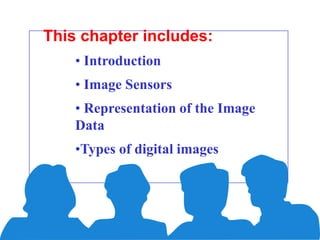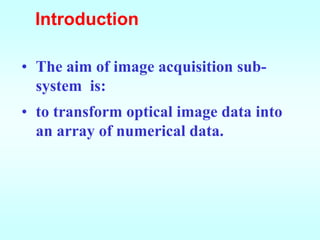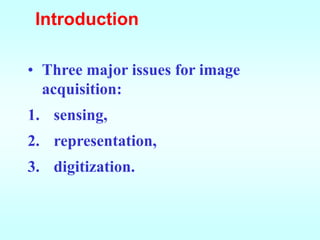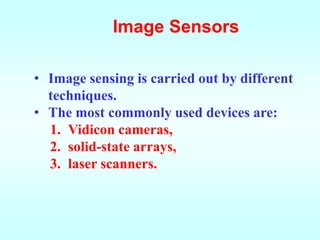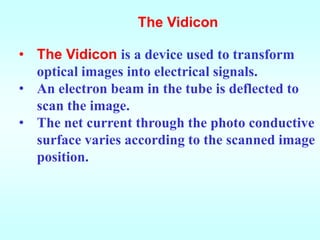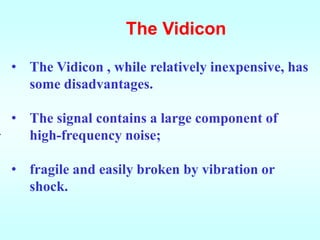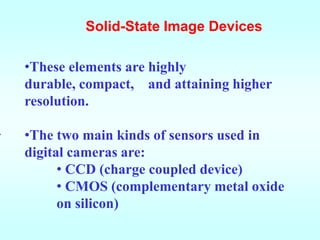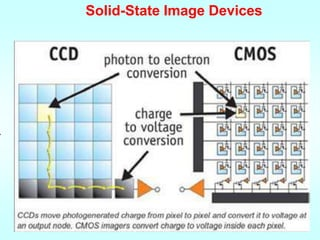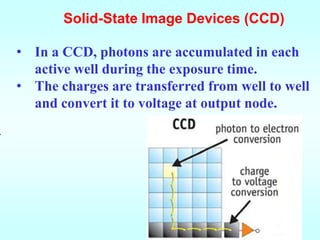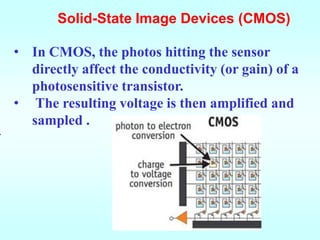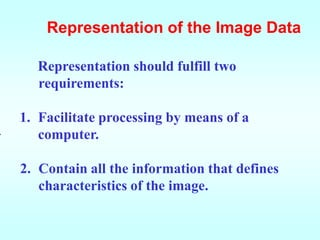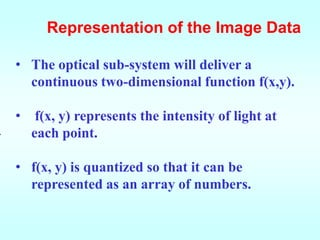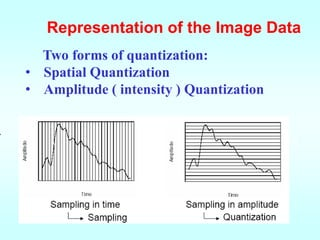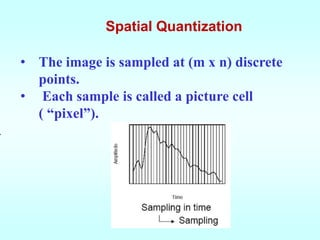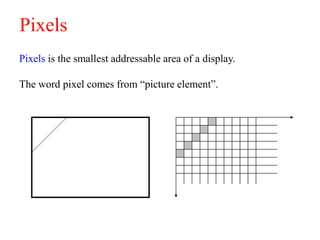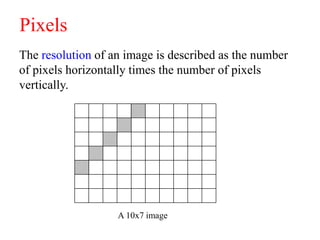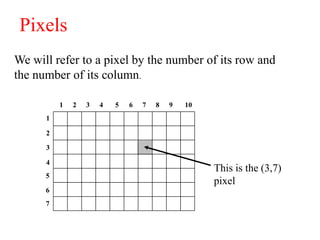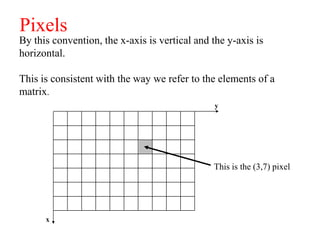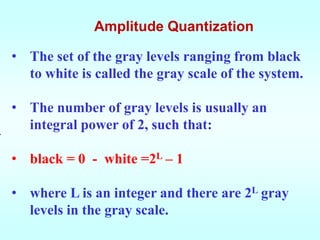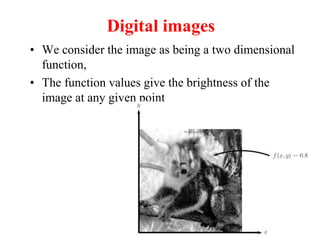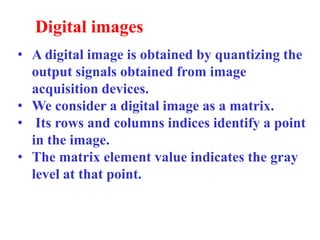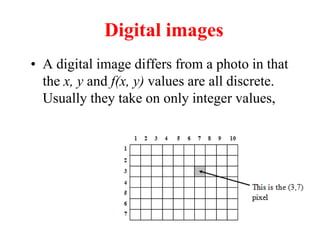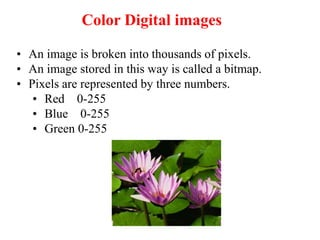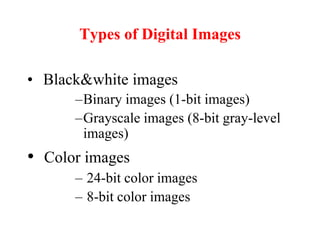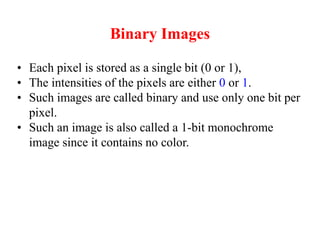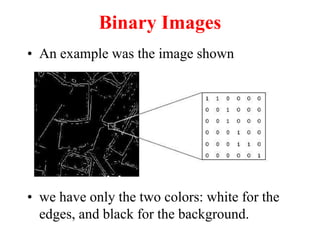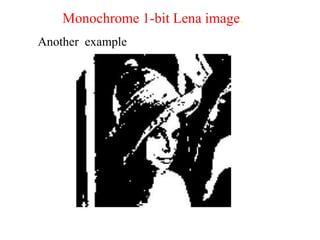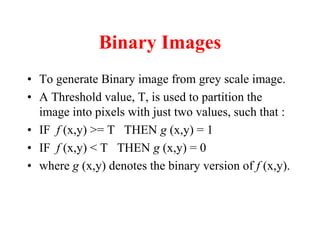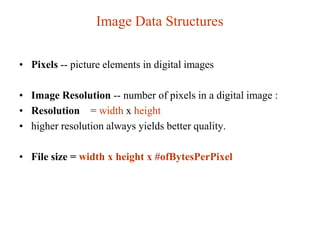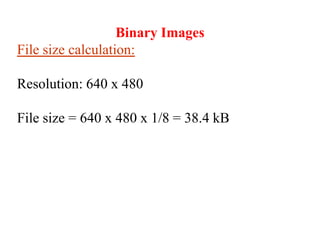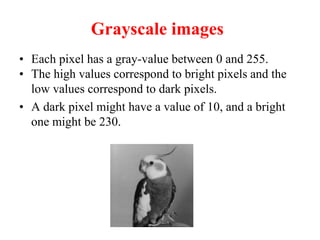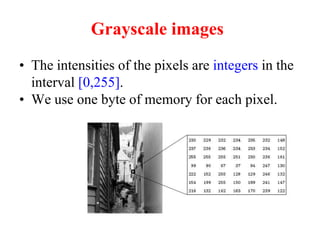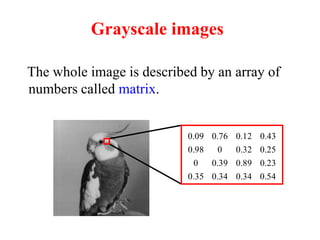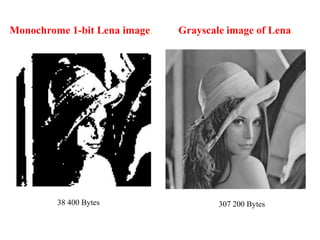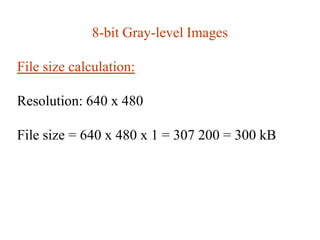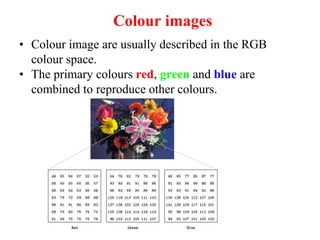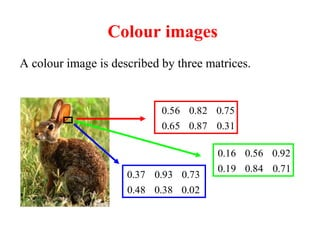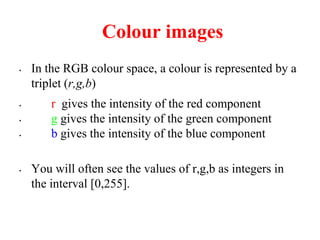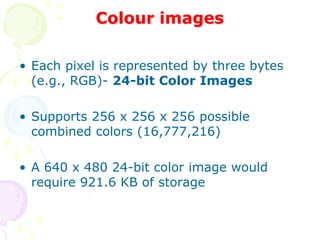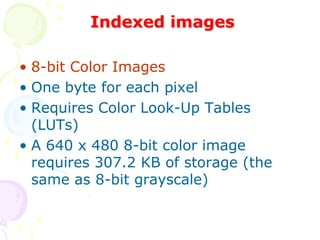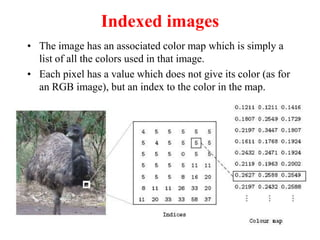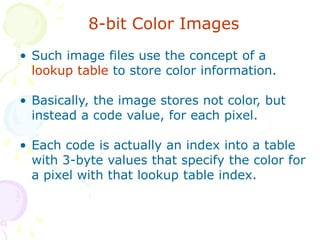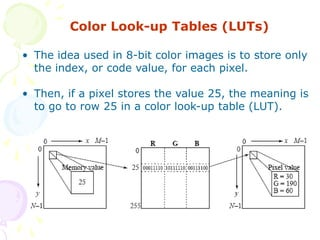1 de 47

### Ch2

• 1. Chapter 2 Image Acquisition
• 2. This chapter includes: • Introduction • Image Sensors • Representation of the Image Data •Types of digital images
• 3. Introduction • The aim of image acquisition sub- system is: • to transform optical image data into an array of numerical data.
• 4. Introduction • Three major issues for image acquisition: 1. sensing, 2. representation, 3. digitization.
• 5. Image Sensors • Image sensing is carried out by different techniques. • The most commonly used devices are: 1. Vidicon cameras, 2. solid-state arrays, 3. laser scanners.
• 6. The Vidicon • The Vidicon is a device used to transform optical images into electrical signals. • An electron beam in the tube is deflected to scan the image. • The net current through the photo conductive surface varies according to the scanned image position.
• 7. The Vidicon • The Vidicon , while relatively inexpensive, has some disadvantages. • The signal contains a large component of . high-frequency noise; • fragile and easily broken by vibration or shock.
• 8. Solid-State Image Devices •These elements are highly durable, compact, and attaining higher resolution. . •The two main kinds of sensors used in digital cameras are: • CCD (charge coupled device) • CMOS (complementary metal oxide on silicon)
• 9. Solid-State Image Devices . .
• 10. Solid-State Image Devices (CCD) • In a CCD, photons are accumulated in each active well during the exposure time. • The charges are transferred from well to well and convert it to voltage at output node. .
• 11. Solid-State Image Devices (CMOS) • In CMOS, the photos hitting the sensor directly affect the conductivity (or gain) of a photosensitive transistor. • The resulting voltage is then amplified and sampled . .
• 12. Laser Scanner • Particularly important in industrial applications. • devices that obtain a “ depth map “. . • The laser light is transmitted and then measuring the phase of the arriving reflected light.
• 13. Representation of the Image Data Representation should fulfill two requirements: 1. Facilitate processing by means of a . computer. 2. Contain all the information that defines characteristics of the image.
• 14. Representation of the Image Data • The optical sub-system will deliver a continuous two-dimensional function f(x,y). • f(x, y) represents the intensity of light at . each point. • f(x, y) is quantized so that it can be represented as an array of numbers.
• 15. Representation of the Image Data Two forms of quantization: • Spatial Quantization • Amplitude ( intensity ) Quantization .
• 16. Spatial Quantization • The image is sampled at (m x n) discrete points. • Each sample is called a picture cell ( “pixel”). .
• 17. Pixels Pixels is the smallest addressable area of a display. The word pixel comes from “picture element”.
• 18. Pixels The resolution of an image is described as the number of pixels horizontally times the number of pixels vertically. A 10x7 image
• 19. Pixels We will refer to a pixel by the number of its row and the number of its column. 1 2 3 4 5 6 7 8 9 10 1 2 3 4 This is the (3,7) 5 pixel 6 7
• 20. Pixels By this convention, the x-axis is vertical and the y-axis is horizontal. This is consistent with the way we refer to the elements of a matrix. y This is the (3,7) pixel x
• 21. Amplitude Quantization • Each pixel assigned a numerical code. • The code represents the intensity of the image function at that point. • The resolution of the code is determined by the number of quantization levels ( gray . levels ).
• 22. Amplitude Quantization • The set of the gray levels ranging from black to white is called the gray scale of the system. • The number of gray levels is usually an . integral power of 2, such that: • black = 0 - white =2L – 1 • where L is an integer and there are 2L gray levels in the gray scale.
• 23. Digital images • We consider the image as being a two dimensional function, • The function values give the brightness of the image at any given point
• 24. Digital images • A digital image is obtained by quantizing the output signals obtained from image acquisition devices. • We consider a digital image as a matrix. • Its rows and columns indices identify a point in the image. • The matrix element value indicates the gray level at that point.
• 25. Digital images • A digital image differs from a photo in that the x, y and f(x, y) values are all discrete. Usually they take on only integer values,
• 26. Color Digital images • An image is broken into thousands of pixels. • An image stored in this way is called a bitmap. • Pixels are represented by three numbers. • Red 0-255 • Blue 0-255 • Green 0-255
• 27. Types of Digital Images • Black&white images –Binary images (1-bit images) –Grayscale images (8-bit gray-level images) • Color images – 24-bit color images – 8-bit color images
• 28. Binary Images • Each pixel is stored as a single bit (0 or 1), • The intensities of the pixels are either 0 or 1. • Such images are called binary and use only one bit per pixel. • Such an image is also called a 1-bit monochrome image since it contains no color.
• 29. Binary Images • An example was the image shown • we have only the two colors: white for the edges, and black for the background.
• 30. Monochrome 1-bit Lena image. Another example
• 31. Binary Images • To generate Binary image from grey scale image. • A Threshold value, T, is used to partition the image into pixels with just two values, such that : • IF f (x,y) >= T THEN g (x,y) = 1 • IF f (x,y) < T THEN g (x,y) = 0 • where g (x,y) denotes the binary version of f (x,y).
• 32. Image Data Structures • Pixels -- picture elements in digital images • Image Resolution -- number of pixels in a digital image : • Resolution = width x height • higher resolution always yields better quality. • File size = width x height x #ofBytesPerPixel
• 33. Binary Images File size calculation: Resolution: 640 x 480 File size = 640 x 480 x 1/8 = 38.4 kB
• 34. Grayscale images • Each pixel has a gray-value between 0 and 255. • The high values correspond to bright pixels and the low values correspond to dark pixels. • A dark pixel might have a value of 10, and a bright one might be 230.
• 35. Grayscale images • The intensities of the pixels are integers in the interval [0,255]. • We use one byte of memory for each pixel.
• 36. Grayscale images The whole image is described by an array of numbers called matrix. 0.09 0.76 0.12 0.43 0.98 0 0.32 0.25 0 0.39 0.89 0.23 0.35 0.34 0.34 0.54
• 37. Grayscale image of Lena.
• 38. Monochrome 1-bit Lena image. Grayscale image of Lena. 38 400 Bytes 307 200 Bytes
• 39. 8-bit Gray-level Images File size calculation: Resolution: 640 x 480 File size = 640 x 480 x 1 = 307 200 = 300 kB
• 40. Colour images • Colour image are usually described in the RGB colour space. • The primary colours red, green and blue are combined to reproduce other colours.
• 41. Colour images A colour image is described by three matrices. 0.56 0.82 0.75 0.65 0.87 0.31 0.16 0.56 0.92 0.19 0.84 0.71 0.37 0.93 0.73 0.48 0.38 0.02
• 42. Colour images • In the RGB colour space, a colour is represented by a triplet (r,g,b) • r gives the intensity of the red component • g gives the intensity of the green component • b gives the intensity of the blue component • You will often see the values of r,g,b as integers in the interval [0,255].
• 43. Colour images • Each pixel is represented by three bytes (e.g., RGB)- 24-bit Color Images • Supports 256 x 256 x 256 possible combined colors (16,777,216) • A 640 x 480 24-bit color image would require 921.6 KB of storage
• 44. Indexed images • 8-bit Color Images • One byte for each pixel • Requires Color Look-Up Tables (LUTs) • A 640 x 480 8-bit color image requires 307.2 KB of storage (the same as 8-bit grayscale)
• 45. Indexed images • The image has an associated color map which is simply a list of all the colors used in that image. • Each pixel has a value which does not give its color (as for an RGB image), but an index to the color in the map.
• 46. 8-bit Color Images • Such image files use the concept of a lookup table to store color information. • Basically, the image stores not color, but instead a code value, for each pixel. • Each code is actually an index into a table with 3-byte values that specify the color for a pixel with that lookup table index.
• 47. Color Look-up Tables (LUTs) • The idea used in 8-bit color images is to store only the index, or code value, for each pixel. • Then, if a pixel stores the value 25, the meaning is to go to row 25 in a color look-up table (LUT).